# Console Application Input Output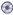Download CD Content

In the previous chapter, we spent some time putting together a very simple Console application that displayed 'Hello World!' when executed. We take what we learned in the example, and apply it to a new application that adds numbers together after being input by the user. Then, we add the ability to calculate the sine of a number to show the basics of the trigonometry functions being used in VB .NET.

 Note The source code for the projects are located on the CD-ROM in the PROJECTS folder. You can either type them in as you go or you can copy the projects from the CD-ROM to your hard drive for editing.

## Getting Started

To start, open Visual Basic and then choose Console Application from the Templates list (see Figure 10.1).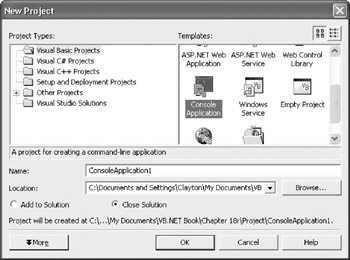Figure 10.1: Choose Console Application from the Templates list.

Next, we're going to add some code to the project.

### Writing Some Code

The first line of code involves adding the Imports System.Console line to the application. This line must be present before Module Module1; your code should look like the following listing after you enter it:

```Imports System.Console
Module Module1

Sub Main()

End Sub

End Module```

Next, we need to declare a couple of variables for the application:

`Dim X,Y As Double`

These variables are used to store the values that are being input by the user and should be placed after the Sub Main() line in the Code Editor. The code should look like the following list:

```Imports System.Console
Module Module1

Sub Main()
Dim X, Y As Double
End Sub

End Module```

Now, we're at a point to begin handling the input, but first, we need to let the user know that we are requesting information from them. We'll begin by using a Write() method to output text information, such as 'Please Enter First Number:'. Instead of WriteLine(), which actually creates an entire line with a carriage return, we'll use Write() so that we can leave our cursor immediately beyond the end of the line for the user input.

Here is the code with the Write() method added:

```Imports System.Console
Module Module1

Sub Main()
Dim X, Y As Double
End Sub

End Module```

Next, we're ready to handle the input from the user and store it in variable X. We can use the ReadLine() method to handle this input:

```Imports System.Console
Module Module1

Sub Main()
Dim X, Y As Double
End Sub

End Module```

We can repeat this same approach for output and input for variable Y:

```Imports System.Console
Module Module1

Sub Main()
Dim X, Y As Double
End Sub

End Module```

The final step for this section of the code is to add the numbers together and then display the output using the Write() and WriteLine() methods. We're using both methods because we'll use Write() to display text such as 'Your final answer is:', and then we'll use WriteLine() to actually display the answer:

```Imports System.Console
Module Module1

Sub Main()
Dim X, Y As Double
WriteLine(X + Y)
End Sub

End Module```

If you run the application using Debug | Start Without Debugging, you'll see output similar to Figure 10.2.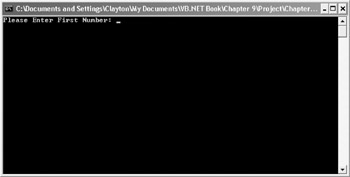Figure 10.2: The code being executed.

We can check the input and output by entering values as prompted. Provide a value of 10 and then press the Enter key on the keyboard. Your window should now look like Figure 10.3.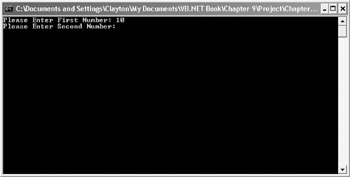Enter a value of 20 and then press the Enter key. This moves the program through the input, and then it displays the final answer of 30, as seen in Figure 10.4.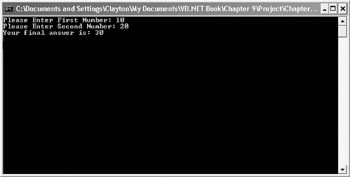Figure 10.4: The answer is displayed.

Because we used a Double as the type of variable, you can also use decimal point values in the program. Try the following combinations:

X: -1.5

Y: 1.5

X: 1.2345

Y: -55.3433

X: 3.75

Y: 4.25

The values should all work equally and aren't dependent on positive or negative values.

## Trigonometry Calculations

The final step in this program is to add one additional calculation. This time, we'll leave the addition operation as is, but we're going to add input and output capabilities to calculate the sine of a number.

This requires the same ReadLine(), Write(), and WriteLine() methods that we used before:

```Imports System.Console
Module Module1

Sub Main()
Dim X, Y As Double
WriteLine(X + Y)

WriteLine()
Write("Calculate Sine of what number: ")
End Sub

End Module```

Notice that we are utilizing the same variable of X for reading the number. The next step, then, is to take the value stored in X and assign X equal to the sine of the value using the Sin method:

```Imports System.Console
Module Module1

Sub Main()
Dim X, Y As Double
WriteLine(X + Y)

WriteLine()
Write("Calculate Sine of what number: ")
X = System.Math.Sin(X)
End Sub

End Module```

Lastly, we'll use the Write() and WriteLine() methods to display the output:

```Imports System.Console
Module Module1

Sub Main()
Dim X, Y As Double
WriteLine(X + Y)

WriteLine()
Write("Calculate Sine of what number: ")
X = System.Math.Sin(X)
Write("The Sine is: ")
WriteLine(X)
End Sub

End Module```

If you run the program at this time, it prompts you for the first two values and then it displays the result after they have been added together. Then, after the calculation, it prompts you to enter another value. The program then outputs the sine of the value (see Figure 10.5).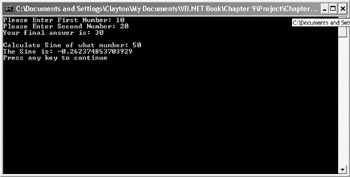Figure 10.5: The final program is being executed.

## Final Code Listing

This is the final code listing for the chapter:

```Imports System.Console
Module Module1

Sub Main()
Dim X, Y As Double
WriteLine(X + Y)

WriteLine()
Write("Calculate Sine of what number: ")
X = System.Math.Sin(X)
Write("The Sine is: ")
WriteLine(X)
End Sub

End Module```

## Summary

In this chapter, we created another Console application; however, unlike the previous chapter, we responded to user input, and then made a few simple calculations based on the values that were entered. We used the ReadLine() method of the System.Console class to capture the input values, and the Sin() method of the System.Math class to calculate the sine of the number that was being entered. In Chapter 11, Your First Windows Forms Application, we build our first Windows Forms application.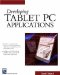Developing Tablet PC Applications (Charles River Media Programming)
ISBN: 1584502525
EAN: 2147483647
Year: 2003
Pages: 191